# 五、基于导数的图像增强

• 图像导数梯度，拉普拉斯
• 锐化和去锐化遮罩（带 PIL，scikit-image、SciPyndimage
• 使用导数和滤波器进行边缘检测（Sobel、Canny、LOG、DOG 等，使用 PIL，scikit-image
• 图像金字塔（高斯和拉普拉斯）-混合图像（带scikit-image

# 图像导数-梯度和拉普拉斯

import numpy as npfrom scipy import signal, misc, ndimagefrom skimage import filters, feature, img_as_floatfrom skimage.io import imreadfrom skimage.color import rgb2grayfrom PIL import Image, ImageFilterimport matplotlib.pylab as pylab


# 导数和梯度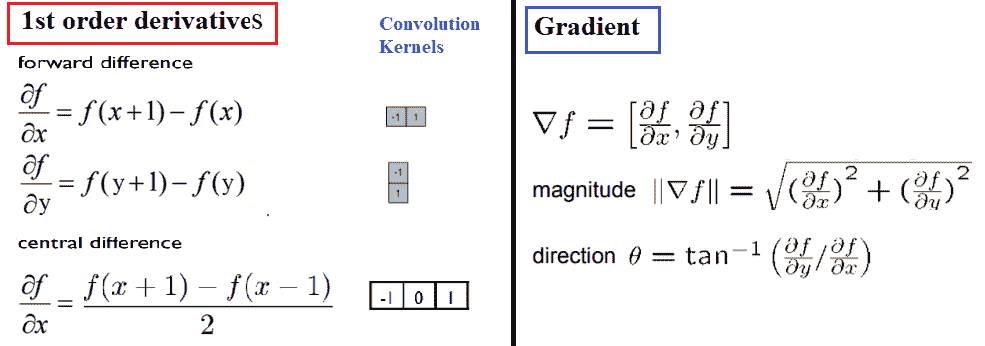def plot_image(image, title):
pylab.imshow(image), pylab.title(title, size=20), pylab.axis('off')

ker_x = [[-1, 1]]
ker_y = [[-1], ]
im_x = signal.convolve2d(im, ker_x, mode='same')
im_y = signal.convolve2d(im, ker_y, mode='same')
im_mag = np.sqrt(im_x**2 + im_y**2)
im_dir = np.arctan(im_y/im_x)
pylab.gray()
pylab.figure(figsize=(30,20))
pylab.subplot(231), plot_image(im, 'original'), pylab.subplot(232), plot_image(im_x, 'grad_x')
pylab.subplot(235), plot_image(im_dir, r'$\theta$'), pylab.subplot(236)
pylab.plot(range(im.shape), im[0,:], 'b-', label=r'$f(x,y)|_{x=0}$', linewidth=5)
pylab.plot(range(im.shape), im_x[0,:], 'r-', label=r'$grad_x (f(x,y))|_{x=0}$')
pylab.title(r'$grad_x (f(x,y))|_{x=0}$', size=30)
pylab.legend(prop={'size': 20})
pylab.show()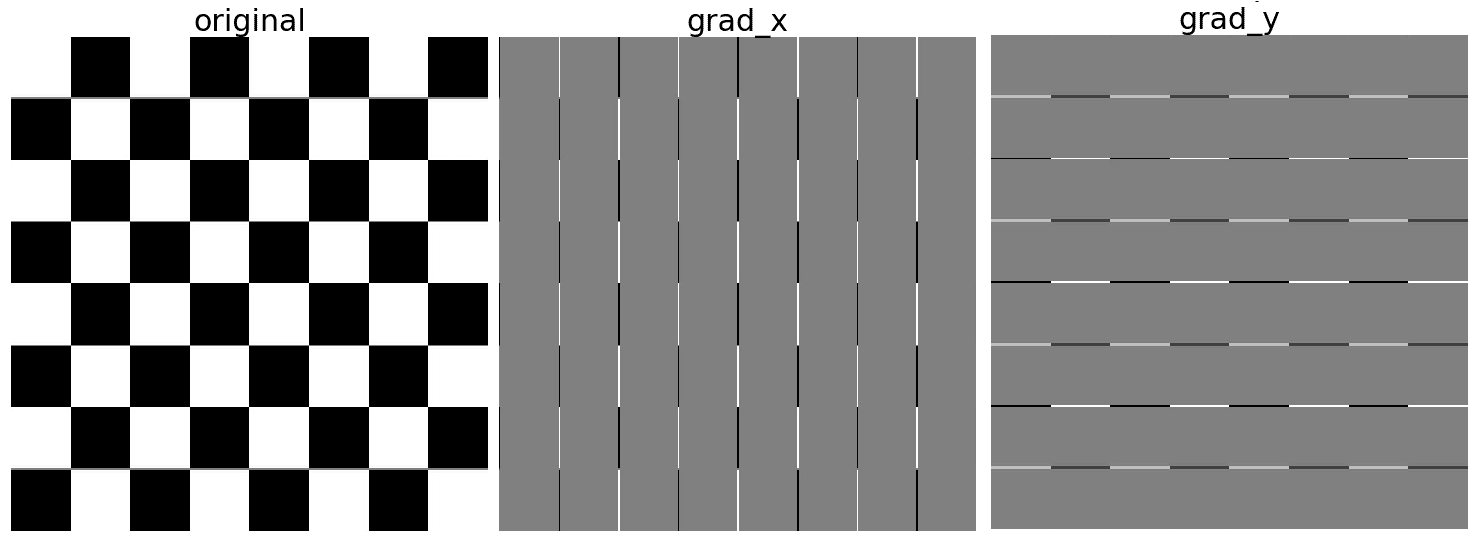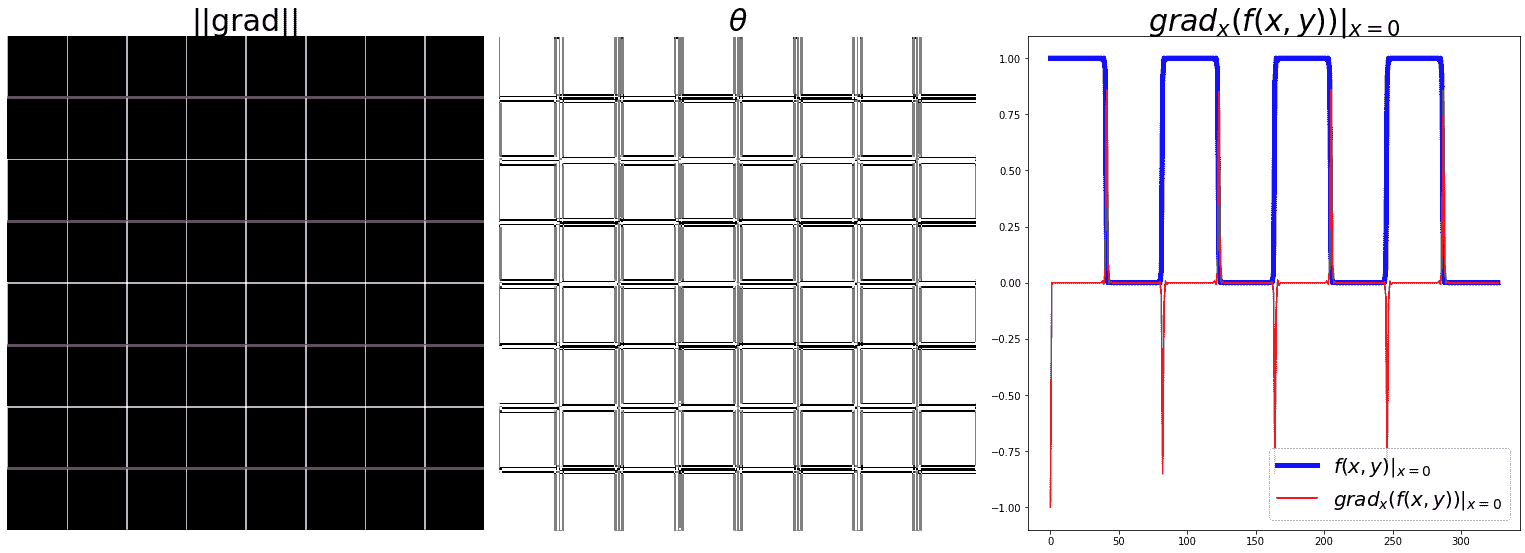# 在同一图像上显示幅值和渐变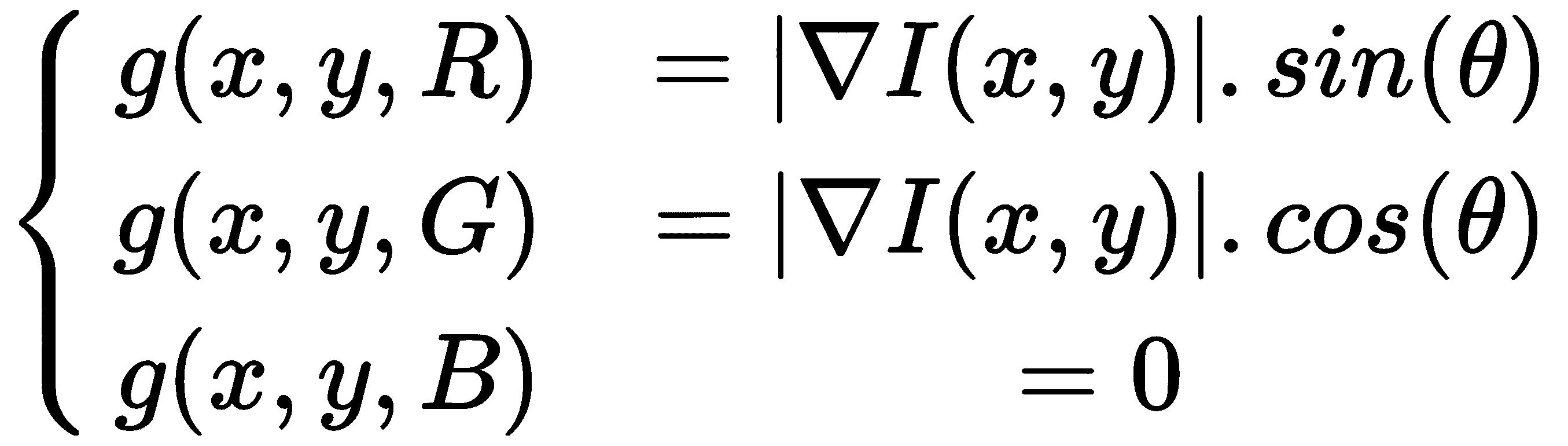im = np.zeros((im.shape,im.shape,3))im[...,0] = im_mag*np.sin(im_ang)im[...,1] = im_mag*np.cos(im_ang)pylab.title(r'||grad||+$\theta$', size=30), pylab.imshow(im), pylab.axis('off')


# 拉普拉斯的

Rosenfeld 和 Kak 已经证明，最简单的各向同性导数算子是拉普拉斯算子，其定义如下图所示。拉普拉斯算子逼近图像的二阶导数并检测边缘。它是各向同性（旋转不变）算子，零交叉标记边缘位置；我们将在本章后面讨论更多关于这一点的内容。换句话说，在输入图像的一阶导数有尖峰/尖峰（或低谷）的位置，在输入图像的二阶导数的相应位置有过零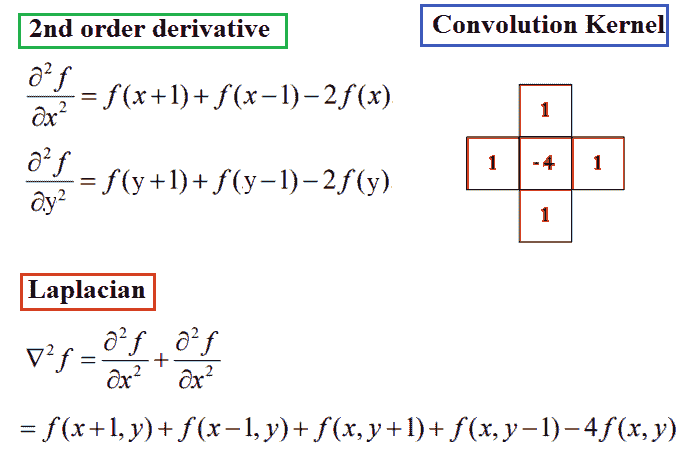# 关于拉普拉斯算子的几点注记

•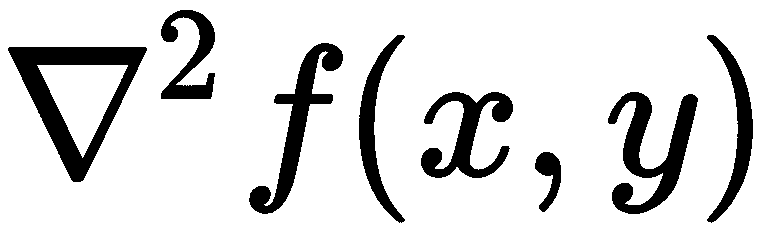是一个标量（与梯度不同，梯度是一个向量）
• 使用单个核（掩码）计算拉普拉斯函数（与梯度不同，梯度通常有两个核，xy方向的偏导数）
• 作为标量，它没有任何方向，因此我们会丢失方向信息
•二阶偏导数的和（梯度表示由一阶偏导数组成的向量），但较高的。。。

# 噪声对梯度计算的影响

from skimage.util import random_noise
sigma = 1 # sd of noise to be added
im = im + random_noise(im, var=sigma**2)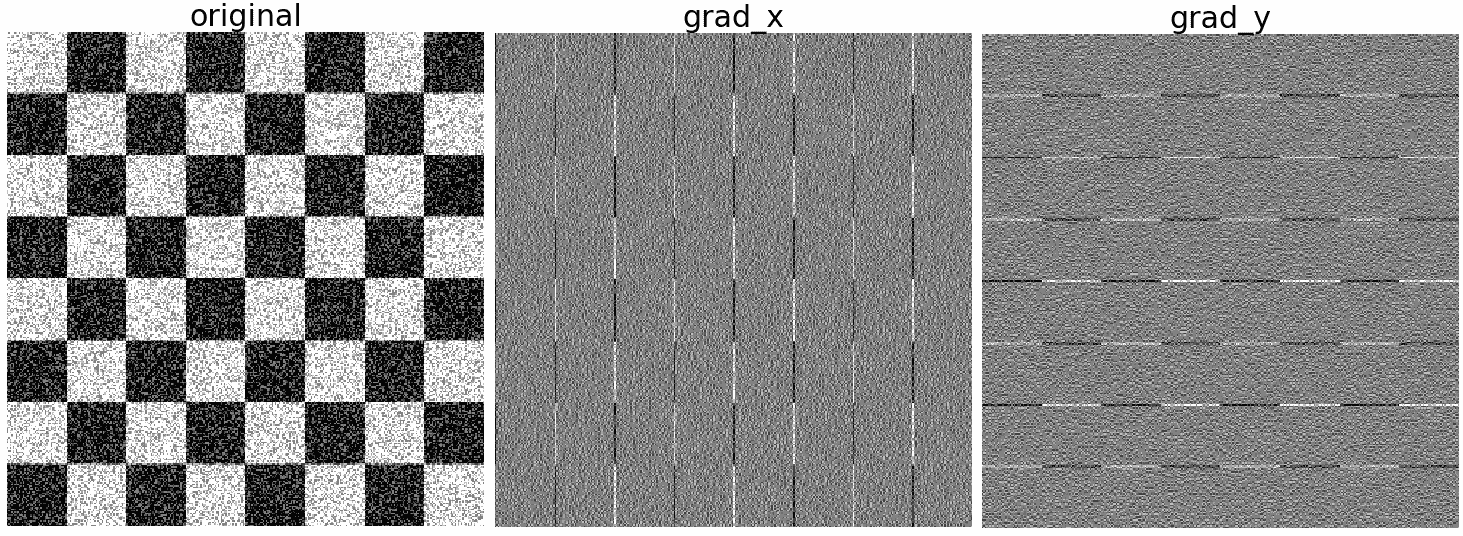# 拉普拉斯锐化

1. 将拉普拉斯滤波器应用于原始输入图像。
2. 步骤 1获得的输出图像与原始输入图像相加（以获得锐化图像）。下面的代码块演示了如何使用scikit-imagefilters模块的laplace()功能实现上述算法：
from skimage.filters import laplace
im1 = np.clip(laplace(im) + im, 0, 1)
pylab.figure(figsize=(20,30))
pylab.subplot(211), plot_image(im, 'original image')
pylab.subplot(212), plot_image(im1, 'sharpened image')
pylab.tight_layout()
pylab.show()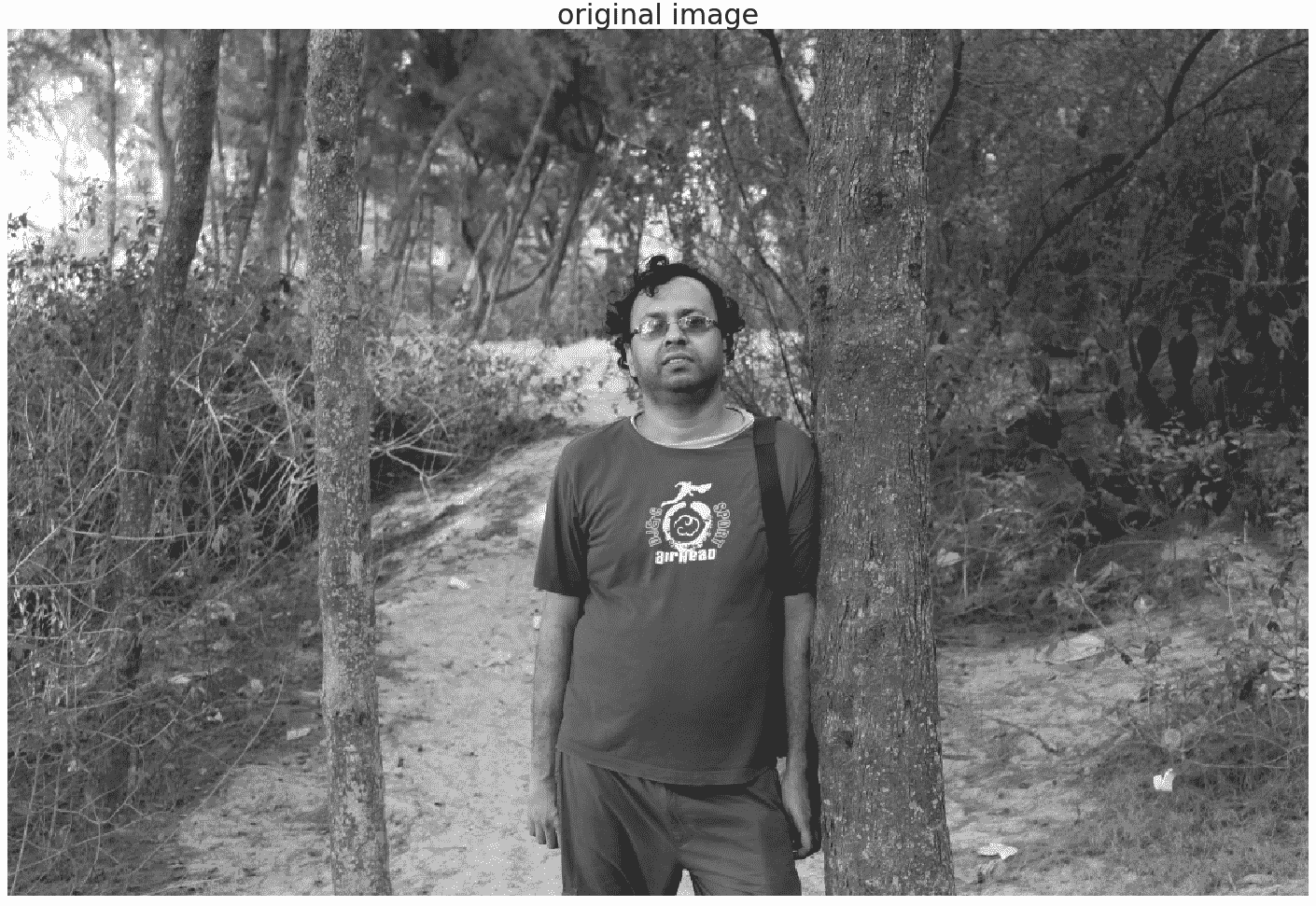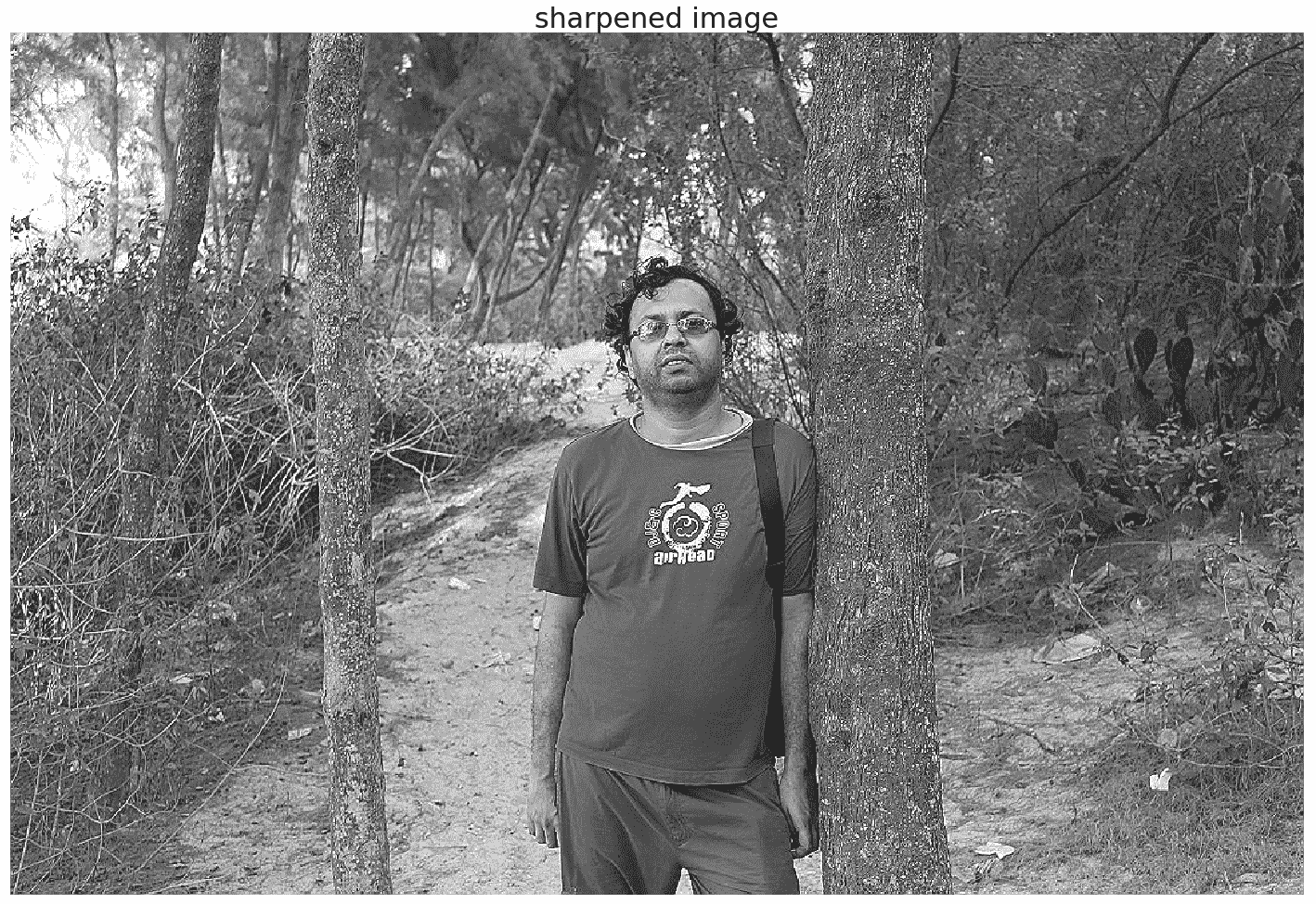# 使用 SciPy ndimage 模块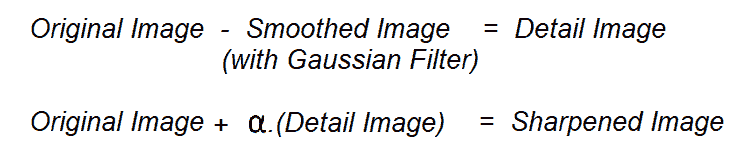def rgb2gray(im):
'''
the input image is an RGB image
with pixel values for each channel in [0,1]
'''
return np.clip(0.2989 * im[...,0] + 0.5870 * im[...,1] + 0.1140 * im[...,2], 0, 1)

im_blurred = ndimage.gaussian_filter(im, 5)
im_detail = np.clip(im - im_blurred, 0, 1)
pylab.gray()
fig, axes = pylab.subplots(nrows=2, ncols=3, sharex=True, sharey=True, figsize=(15, 15))
axes = axes.ravel()
axes.set_title('Original image', size=15), axes.imshow(im)
axes.set_title('Blurred image, sigma=5', size=15), axes.imshow(im_blurred)
axes.set_title('Detail image', size=15), axes.imshow(im_detail)
alpha = [1, 5, 10]
for i in range(3):
im_sharp = np.clip(im + alpha[i]*im_detail, 0, 1)
axes[3+i].imshow(im_sharp),  axes[3+i].set_title('Sharpened image, alpha=' + str(alpha[i]), size=15)
for ax in axes:
ax.axis('off')
fig.tight_layout()
pylab.show()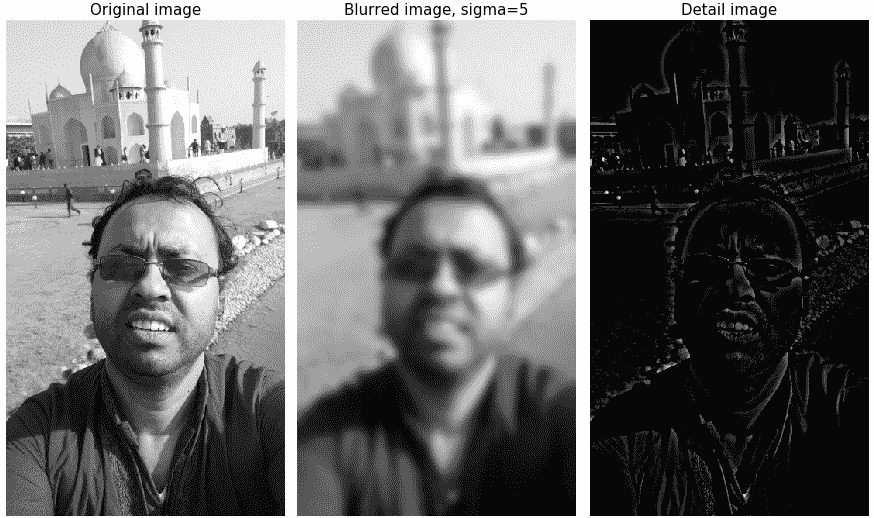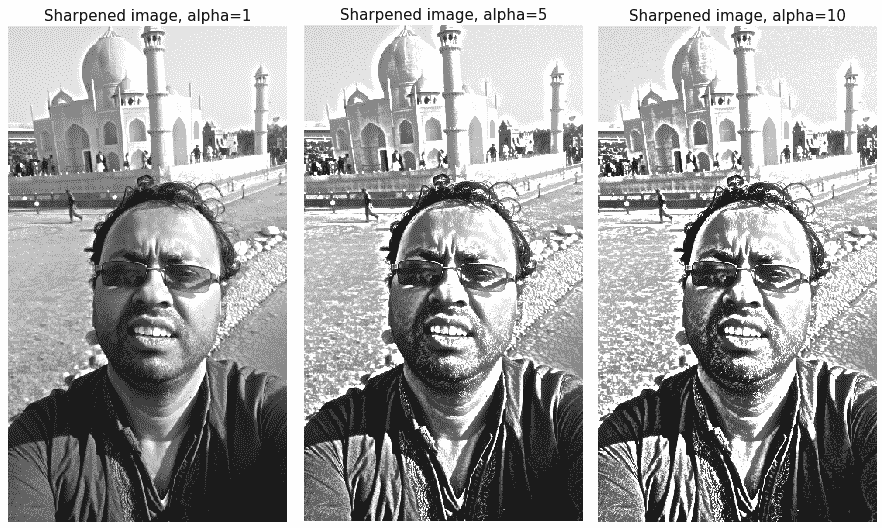# 使用偏导数计算梯度幅值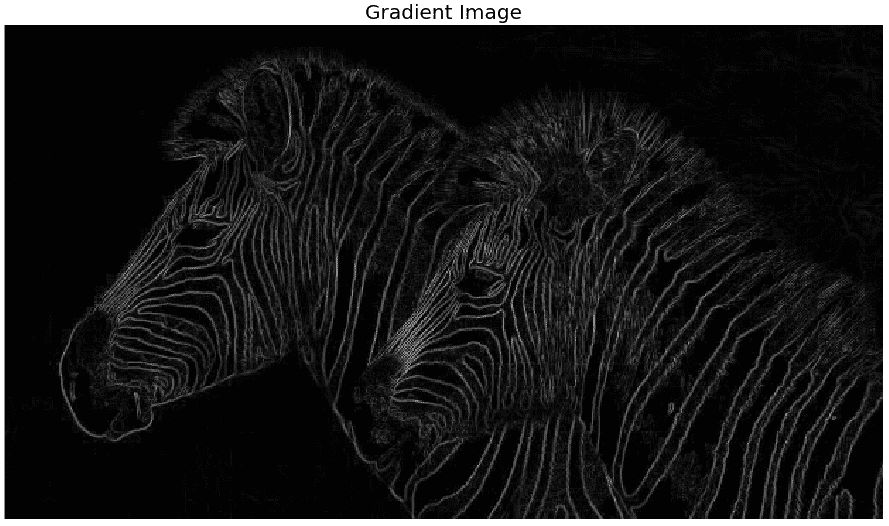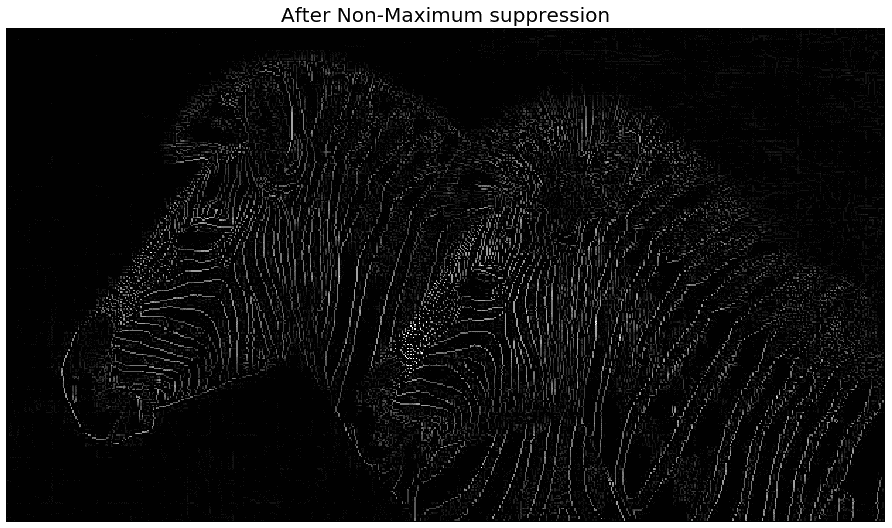# 非最大值抑制算法

1. 该算法首先检查边缘的角度（方向）（由边缘检测器输出）。
2. 如果像素值在与其边缘角度相切的直线上不是最大值，则该像素值是要从边缘贴图中删除的候选像素。
3. 这是通过将边缘方向（360）拆分为八个相等的间隔（角度为 22.50 度）来实现的。下表显示了不同的情况和要采取的措施：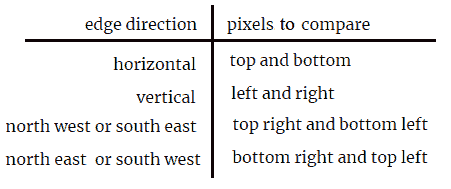1. 我们可以通过观察π/8 范围并相应地设置一系列 if 条件的切向比较来实现这一点。
2. 可以清楚地观察到边缘细化的效果（从上一张图像中）。。。

# 基于 scikit 图像的 Sobel 边缘检测器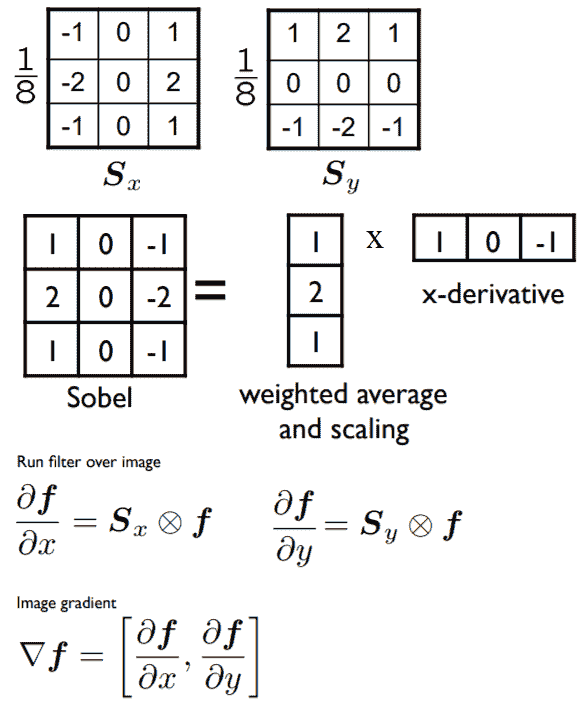Sobel 算子的标准定义中不包括 1/8 项，因为出于边缘检测的目的，它不会产生差异，尽管需要标准化项才能正确获得梯度值。下一个 Python 代码片段展示了如何分别使用scikit-imagefilters模块的sobel_h()sobel_y()sobel()函数来查找水平/垂直边缘，并使用 Sobel 运算符计算梯度大小：

im = rgb2gray(imread('../images/tajmahal.jpg')) # RGB image to gray scale
pylab.gray()
pylab.figure(figsize=(20,18))
pylab.subplot(2,2,1)
plot_image(im, 'original')
pylab.subplot(2,2,2)
edges_x = filters.sobel_h(im)
plot_image(edges_x, 'sobel_x')
pylab.subplot(2,2,3)
edges_y = filters.sobel_v(im)
plot_image(edges_y, 'sobel_y')
pylab.subplot(2,2,4)
edges = filters.sobel(im)
plot_image(edges, 'sobel')
pylab.show()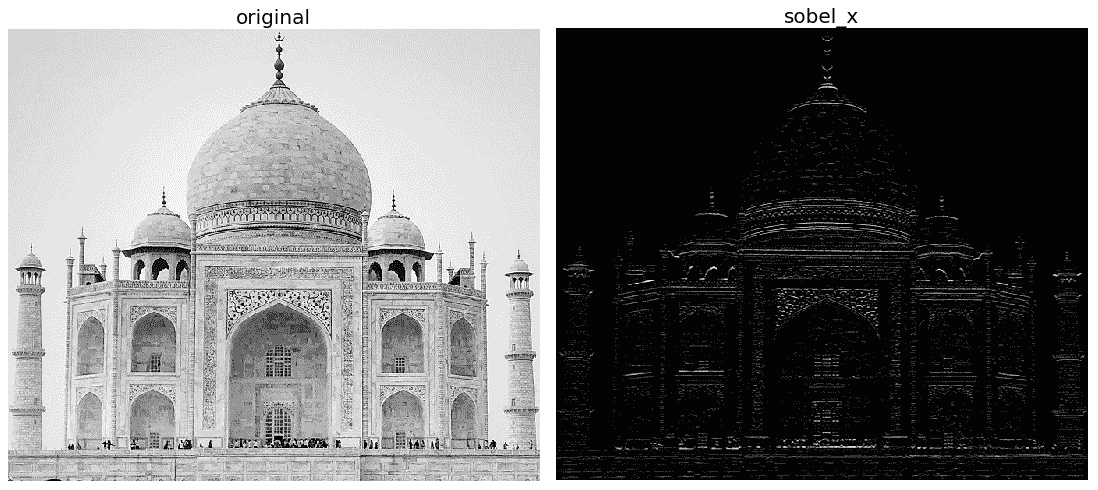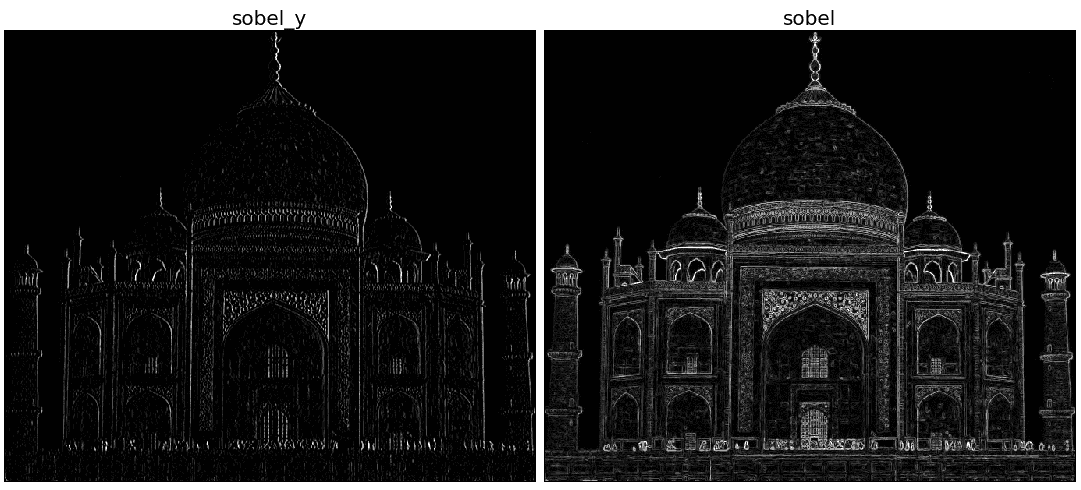# 具有 scikit 图像的不同边缘检测器–Prewitt、Roberts、Sobel、Scharr 和 Laplace

scikit-image文件中所述。。。

# 基于 scikit 图像的 Canny 边缘检测器

Canny 边缘检测器是一种流行的边缘检测算法，由 John F.Canny 开发。此算法具有以下多个步骤：

1. 平滑/降噪：边缘检测操作对噪声敏感。因此，在一开始，使用 5 x 5 高斯滤波器从图像中去除噪声。
2. 计算梯度的大小和方向：然后对图像应用 Sobel 水平和垂直滤波器，以计算每个像素的边缘梯度大小方向，如前所述。然后将计算出的梯度角（方向）四舍五入为表示每个像素的水平、垂直和两个对角线方向的四个角之一。
3. 非最大抑制：在这一步中，边缘被减薄–可能不构成边缘的任何不需要的像素被移除。要做到这一点，每个像素都要检查它是否是其邻域中梯度方向上的局部最大值。结果，获得具有薄边缘的二值图像。

4. 链接和滞后阈值：此步骤决定检测到的所有边缘是否都是强边缘。为此，使用了一对（滞后）阈值min_valmax_val。确定边缘是强度梯度值高于max_val的边缘。确保非边是强度梯度值低于min_val的边，它们将被丢弃。位于这两个阈值之间的边根据其连通性分类为边或非边。如果它们连接到“确定边缘”像素，则它们被视为边缘的一部分。否则，它们也会被丢弃。这一步还去除了小像素噪声（假设边缘是长线）。

im = rgb2gray(imread('../images/tiger3.jpg'))
im = ndimage.gaussian_filter(im, 4)
im += 0.05 * np.random.random(im.shape)
edges1 = feature.canny(im)
edges2 = feature.canny(im, sigma=3)
fig, (axes1, axes2, axes3) = pylab.subplots(nrows=1, ncols=3, figsize=(30, 12), sharex=True, sharey=True)
axes1.imshow(im, cmap=pylab.cm.gray), axes1.axis('off'), axes1.set_title('noisy image', fontsize=50)
axes2.imshow(edges1, cmap=pylab.cm.gray), axes2.axis('off')
axes2.set_title('Canny filter, $\sigma=1$', fontsize=50)
axes3.imshow(edges2, cmap=pylab.cm.gray), axes3.axis('off')
axes3.set_title('Canny filter, $\sigma=3$', fontsize=50)
fig.tight_layout()
pylab.show()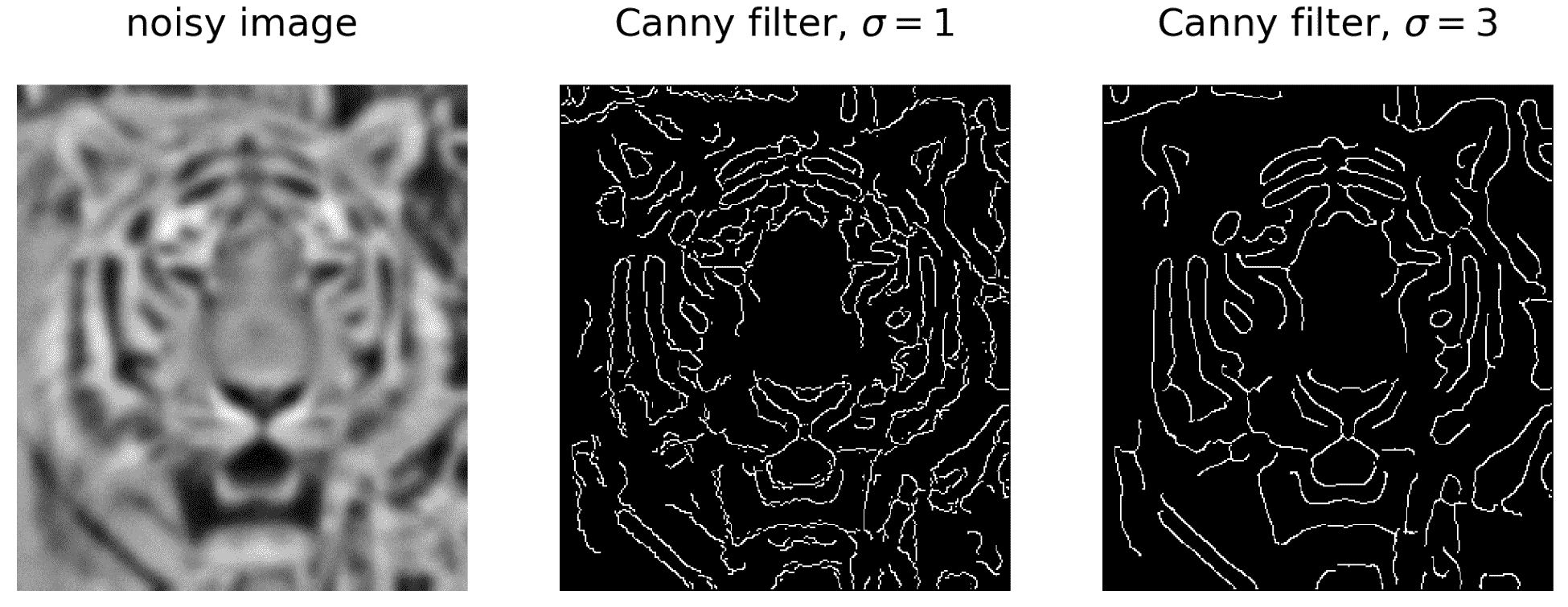# 带有 SciPy ndimage 模块的日志过滤器

SciPyndimage模块的gaussian_laplace()功能也可以用来实现日志，如下代码块所示：

img = rgb2gray(imread('../images/zebras.jpg'))
fig = pylab.figure(figsize=(25,15))
pylab.gray() # show the filtered result in grayscale
for sigma in range(1,10):
pylab.subplot(3,3,sigma)
img_log = ndimage.gaussian_laplace(img, sigma=sigma)
pylab.imshow(np.clip(img_log,0,1)), pylab.axis('off')
pylab.title('LoG with sigma=' + str(sigma), size=20)
pylab.show()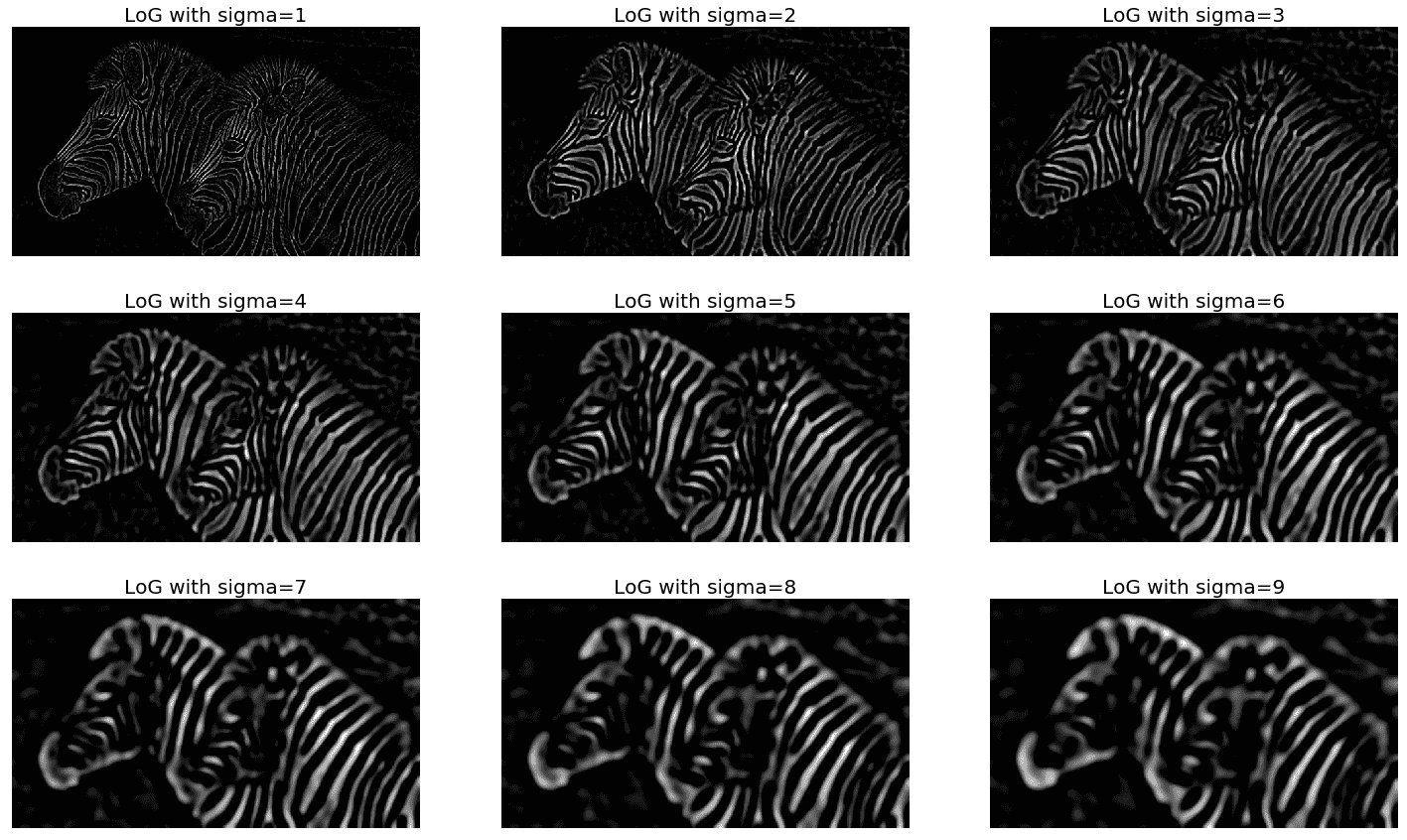# 基于对数滤波器的边缘检测

• 首先，输入图像需要平滑（通过与高斯滤波器卷积）。
• 然后，平滑后的图像需要用拉普拉斯滤波器进行卷积，得到输出图像为2（I（x，y）G（x，y））*。

• 最后，需要计算在最后一步中获得的图像的过零点，如下图所示：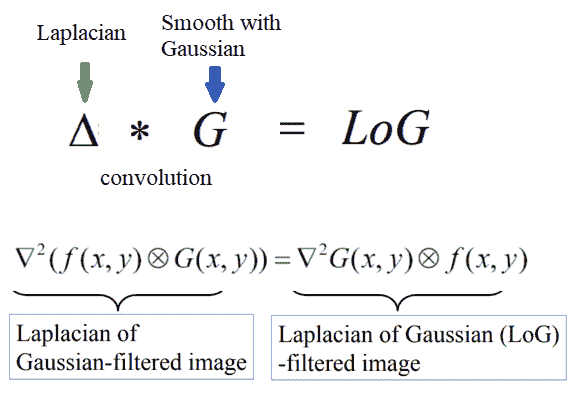# 基于过零计算的 Marr 和 Hildreth 边缘检测算法

Marr 和 Hildreth 提出了计算对数卷积图像中的过零点（将边缘检测为二值图像）。通过将对数平滑的图像定义为二值图像来查看其符号，可以识别边缘像素。计算过零的算法如下：

1. 首先，将对数卷积图像转换为二值图像，将像素值替换为1表示正值，将0表示负值
2. 为了计算过零像素，我们只需查看此二值图像中非零区域的边界
3. 边界可以通过查找任何非零像素来找到，该像素的近邻为零
4. 因此，对于每个像素，如果它是非零的，考虑它的八个邻居；如果任何相邻像素为零，则该像素可被识别为边缘

fig = pylab.figure(figsize=(25,15))
pylab.gray() # show the filtered result in grayscale
for sigma in range(2,10, 2):
pylab.subplot(2,2,sigma/2)
result = ndimage.gaussian_laplace(img, sigma=sigma)
pylab.imshow(zero_crossing(result)) # implement the function zero_crossing() using the above algorithm
pylab.axis('off')
pylab.title('LoG with zero-crossing, sigma=' + str(sigma), size=20)
pylab.show()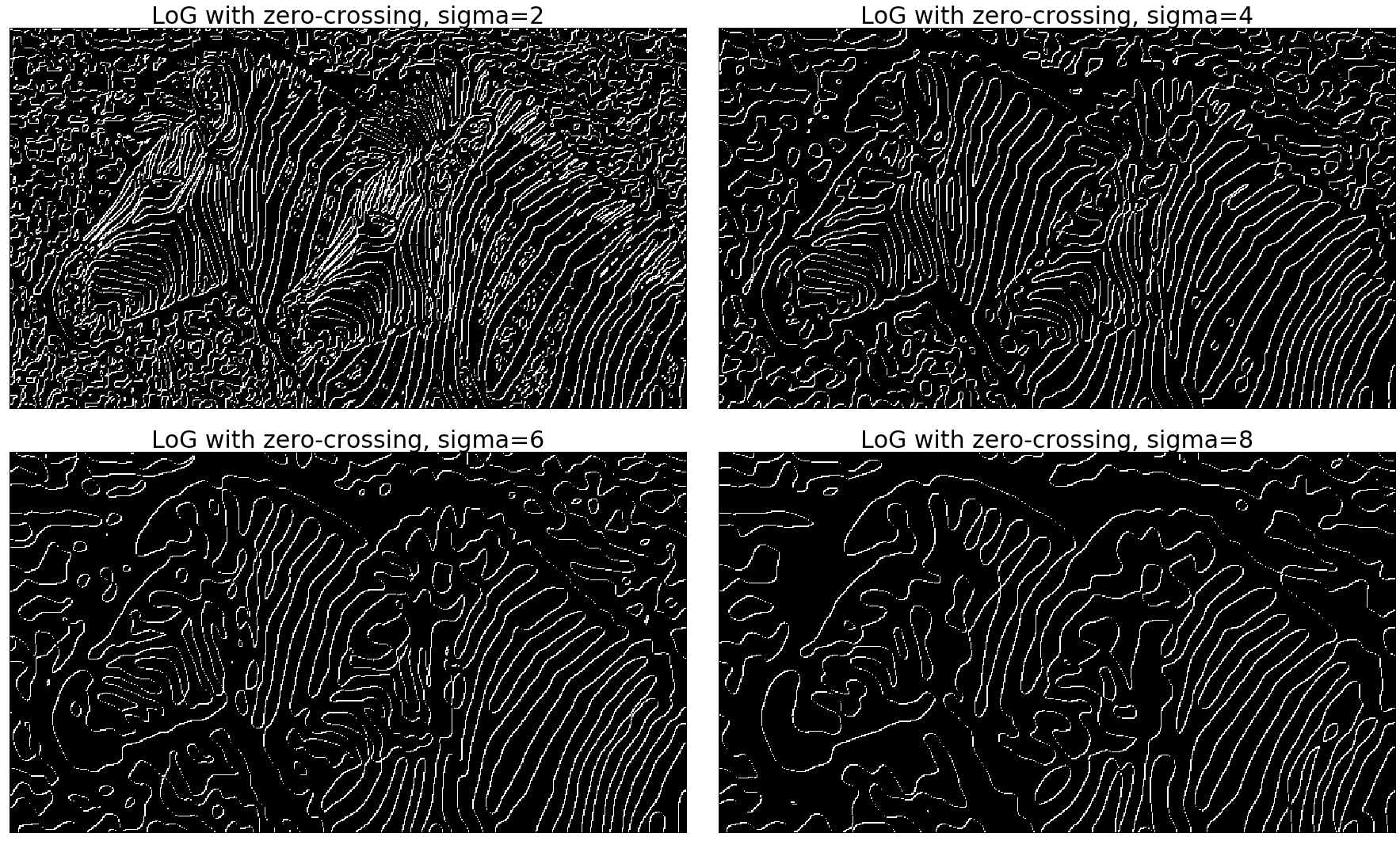# 用 PIL 查找和增强边缘

PIL 的ImageFilter模块的filter功能也可用于查找和增强图像中的边缘。以下代码块显示了以UMBC library图像作为输入的示例：

from PIL.ImageFilter import (FIND_EDGES, EDGE_ENHANCE, EDGE_ENHANCE_MORE)im = Image.open('../images/umbc_lib.jpg')pylab.figure(figsize=(18,25))pylab.subplot(2,2,1)plot_image(im, 'original (UMBC library)')i = 2for f in (FIND_EDGES, EDGE_ENHANCE, EDGE_ENHANCE_MORE): pylab.subplot(2,2,i) im1 = im.filter(f) plot_image(im1, str(f)) i += 1pylab.show()


# 带有 scikit 图像变换金字塔模块的高斯金字塔

from skimage.transform import pyramid_gaussianimage = imread('../images/lena.jpg')nrows, ncols = image.shape[:2]pyramid = tuple(pyramid_gaussian(image, downscale=2))pylab.figure(figsize=(20,5))i, n = 1, len(pyramid)for p in pyramid: pylab.subplot(1,n,i), pylab.imshow(p) pylab.title(str(p.shape) ...


# 具有 scikit 图像变换金字塔模块的拉普拉斯金字塔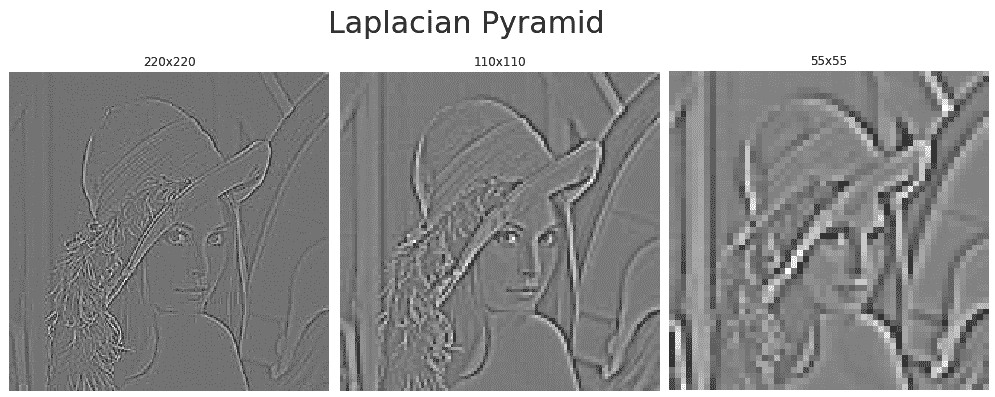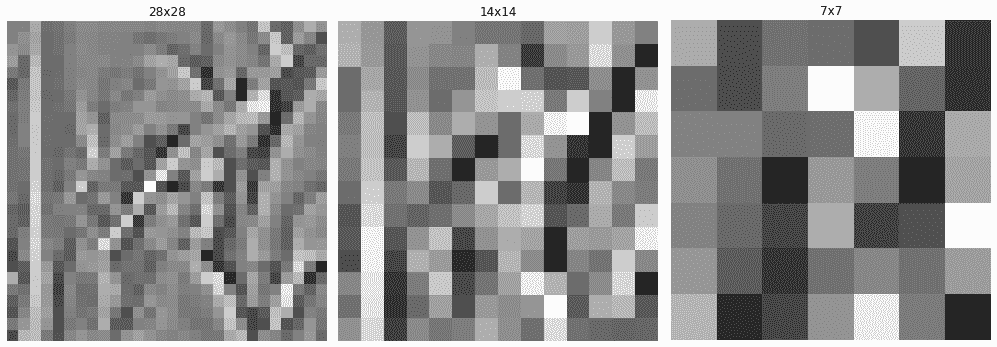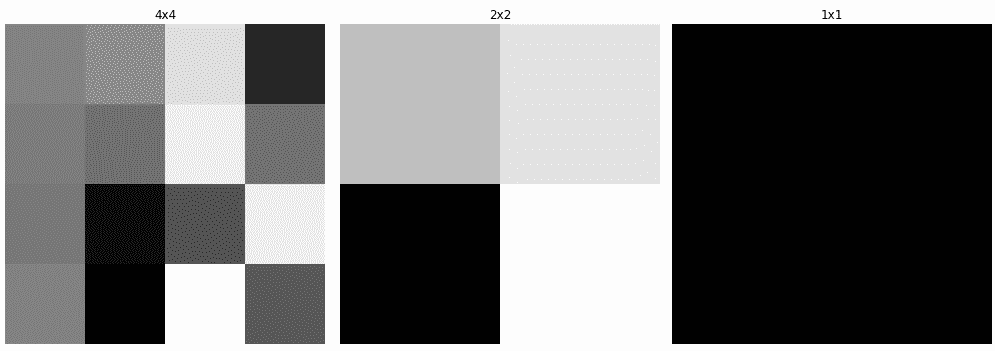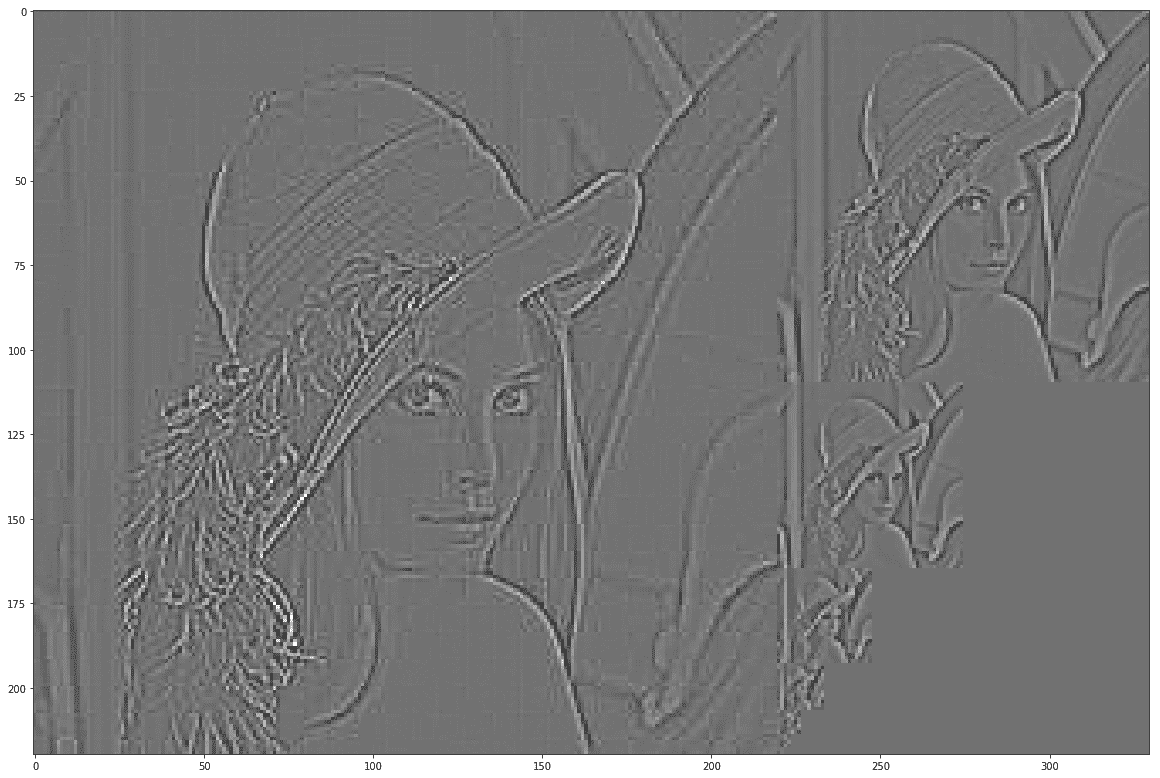# 构造高斯金字塔

1. 从原始图像开始。
2. 在金字塔的每一层迭代计算图像，首先通过平滑图像（使用高斯滤波器），然后对其进行下采样。
3. 在图像大小足够小的级别停止（例如，1 x 1）。
4. 实现前面算法的函数留给读者作为练习；我们只需在以下函数中添加几行即可完成实现：
from skimage.transform import pyramid_reduce def get_gaussian_pyramid(image):     '''    input: an RGB image    output: the Gaussian Pyramid of the image as a list    '''    gaussian_pyramid = []    # add code here # iteratively ...


# 仅从拉普拉斯金字塔重建图像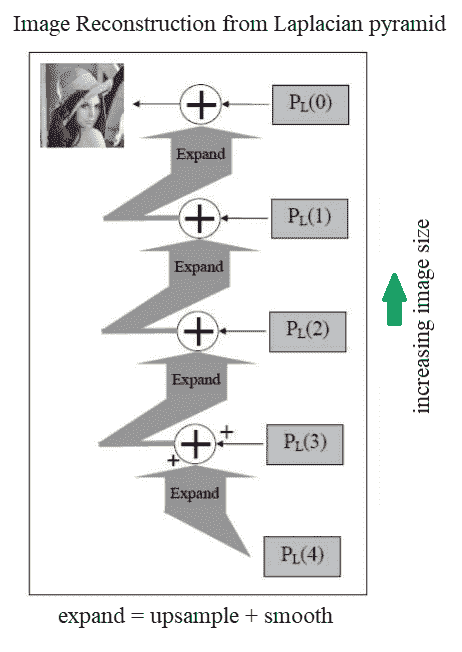def reconstruct_image_from_laplacian_pyramid(pyramid):
i = len(pyramid) - 2
prev = pyramid[i+1]
pylab.figure(figsize=(20,20))
j = 1
while i >= 0:
prev = resize(pyramid_expand(prev, upscale=2), pyramid[i].shape)
im = np.clip(pyramid[i] + prev,0,1)
pylab.subplot(3,3,j), pylab.imshow(im)
pylab.title('Level=' + str(j) + ' ' + str(im.shape) + 'x' + str(im.shape), size=20)
prev = im
i -= 1
j += 1
pylab.subplot(3,3,j), pylab.imshow(image)
pylab.title('Original image' + ' ' + str(image.shape) + 'x' + str(image.shape), size=20)
pylab.show()
return im

image = img_as_float(imread('../images/apple.png')[...,:3]) # only use the color channels and discard the alpha
pyramid = get_laplacian_pyramid(get_gaussian_pyramid(image))
im = reconstruct_image_from_laplacian_pyramid(pyramid)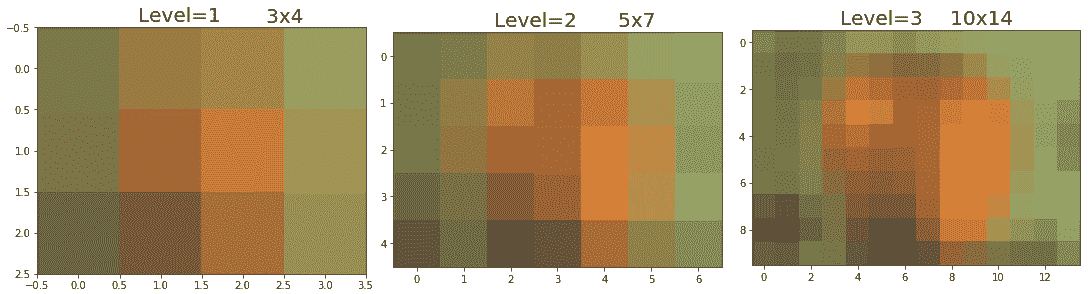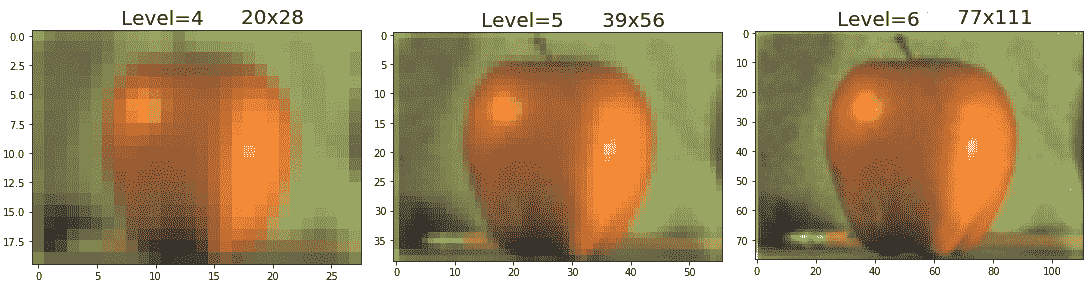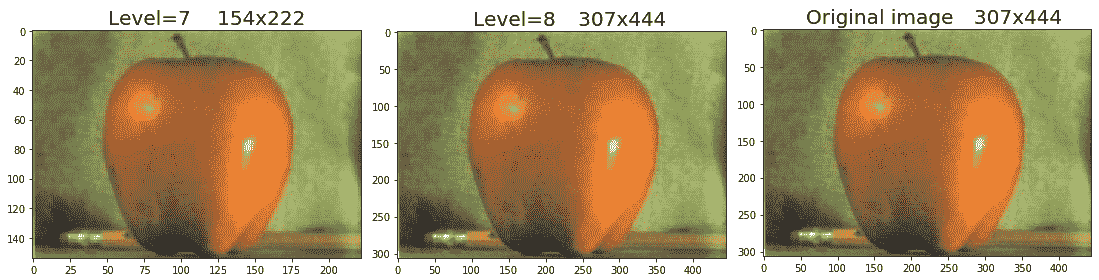# 问题

1. 使用skimage.filters模块的unsharp_mask()功能和radiusamount参数的不同值来锐化图像。
2. 使用 PILImageFilter模块的UnsharpMask()功能以及radiuspercent参数的不同值来锐化图像。
3. 使用锐化内核[[0，-1，0]、-1，5，-1]、[0，-1，0]]锐化彩色（RGB）图像。（提示：对每个颜色通道逐一使用 SciPysignal模块的convolve2d()功能。）
4. 使用 SciPyndimage模块，可直接锐化彩色图像（无需逐个锐化单个颜色通道）。
5. 使用skimage.transform模块的pyramid_laplacian()函数计算并显示带有lena灰度输入图像的高斯金字塔。

6. 建筑